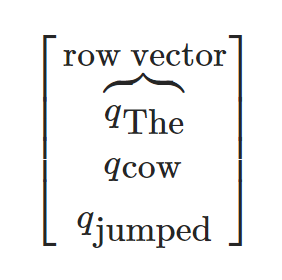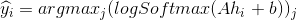# 序列模型和长短句记忆（LSTM）模型

• 前馈网络

• 序列模型

• 循环神经网络

## 1.Pytorch中的LSTM``````# Author: Robert Guthrie

import torch
import torch.nn as nn
import torch.nn.functional as F
import torch.optim as optim

torch.manual_seed(1)
``````
``````lstm = nn.LSTM(3, 3)  # 输入维度为3维，输出维度为3维
inputs = [torch.randn(1, 3) for _ in range(5)]  # 生成一个长度为5的序列

# 初始化隐藏状态.
hidden = (torch.randn(1, 1, 3),
torch.randn(1, 1, 3))
for i in inputs:
# 将序列中的元素逐个输入到LSTM.
# 经过每步操作,hidden 的值包含了隐藏状态的信息.
out, hidden = lstm(i.view(1, 1, -1), hidden)

# 另外我们可以对一整个序列进行训练.
# LSTM第一个返回的第一个值是所有时刻的隐藏状态
# 第二个返回值是最后一个时刻的隐藏状态
#(所以"out"的最后一个和"hidden"是一样的)
# 之所以这样设计:
# 通过"out"你能取得任何一个时刻的隐藏状态，而"hidden"的值是用来进行序列的反向传播运算, 具体方式就是将它作为参数传入后面的 LSTM 网络.

# 增加额外的第二个维度.
inputs = torch.cat(inputs).view(len(inputs), 1, -1)
hidden = (torch.randn(1, 1, 3), torch.randn(1, 1, 3))  # 清空隐藏状态.
out, hidden = lstm(inputs, hidden)
print(out)
print(hidden)
``````
• 输出结果：
``````tensor([[[-0.0187,  0.1713, -0.2944]],

[[-0.3521,  0.1026, -0.2971]],

[[-0.3191,  0.0781, -0.1957]],

[[-0.1634,  0.0941, -0.1637]],

``````

## 2.例子:用LSTM来进行词性标注#### 2.1 准备数据

``````def prepare_sequence(seq, to_ix):
idxs = [to_ix[w] for w in seq]

training_data = [
("The dog ate the apple".split(), ["DET", "NN", "V", "DET", "NN"]),
("Everybody read that book".split(), ["NN", "V", "DET", "NN"])
]
word_to_ix = {}
for sent, tags in training_data:
for word in sent:
if word not in word_to_ix:
word_to_ix[word] = len(word_to_ix)
print(word_to_ix)
tag_to_ix = {"DET": 0, "NN": 1, "V": 2}

# 实际中通常使用更大的维度如32维, 64维.
# 这里我们使用小的维度, 为了方便查看训练过程中权重的变化.
EMBEDDING_DIM = 6
HIDDEN_DIM = 6
``````
• 输出结果：
``````{'The': 0, 'dog': 1, 'ate': 2, 'the': 3, 'apple': 4, 'Everybody': 5, 'read': 6, 'that': 7, 'book': 8}
``````

#### 2.1 创建模型

``````class LSTMTagger(nn.Module):

def __init__(self, embedding_dim, hidden_dim, vocab_size, tagset_size):
super(LSTMTagger, self).__init__()
self.hidden_dim = hidden_dim

self.word_embeddings = nn.Embedding(vocab_size, embedding_dim)

# LSTM以word_embeddings作为输入, 输出维度为 hidden_dim 的隐藏状态值
self.lstm = nn.LSTM(embedding_dim, hidden_dim)

# 线性层将隐藏状态空间映射到标注空间
self.hidden2tag = nn.Linear(hidden_dim, tagset_size)
self.hidden = self.init_hidden()

def init_hidden(self):
# 一开始并没有隐藏状态所以我们要先初始化一个
# 关于维度为什么这么设计请参考Pytoch相关文档
# 各个维度的含义是 (num_layers, minibatch_size, hidden_dim)
return (torch.zeros(1, 1, self.hidden_dim),
torch.zeros(1, 1, self.hidden_dim))

def forward(self, sentence):
embeds = self.word_embeddings(sentence)
lstm_out, self.hidden = self.lstm(
embeds.view(len(sentence), 1, -1), self.hidden)
tag_space = self.hidden2tag(lstm_out.view(len(sentence), -1))
tag_scores = F.log_softmax(tag_space, dim=1)
return tag_scores
``````

#### 2.3 训练模型

``````model = LSTMTagger(EMBEDDING_DIM, HIDDEN_DIM, len(word_to_ix), len(tag_to_ix))
loss_function = nn.NLLLoss()
optimizer = optim.SGD(model.parameters(), lr=0.1)

# 查看训练前的分数
# 注意: 输出的 i,j 元素的值表示单词 i 的 j 标签的得分
inputs = prepare_sequence(training_data, word_to_ix)
tag_scores = model(inputs)
print(tag_scores)

for epoch in range(300):  # 实际情况下你不会训练300个周期, 此例中我们只是随便设了一个值
for sentence, tags in training_data:
# 第一步: 请记住Pytorch会累加梯度.
# 我们需要在训练每个实例前清空梯度

# 此外还需要清空 LSTM 的隐状态,
# 将其从上个实例的历史中分离出来.
model.hidden = model.init_hidden()

# 准备网络输入, 将其变为词索引的 Tensor 类型数据
sentence_in = prepare_sequence(sentence, word_to_ix)
targets = prepare_sequence(tags, tag_to_ix)

# 第三步: 前向传播.
tag_scores = model(sentence_in)

# 第四步: 计算损失和梯度值, 通过调用 optimizer.step() 来更新梯度
loss = loss_function(tag_scores, targets)
loss.backward()
optimizer.step()

# 查看训练后的得分
inputs = prepare_sequence(training_data, word_to_ix)
tag_scores = model(inputs)

# 句子是 "the dog ate the apple", i,j 表示对于单词 i, 标签 j 的得分.
# 我们采用得分最高的标签作为预测的标签. 从下面的输出我们可以看到, 预测得
# 到的结果是0 1 2 0 1. 因为 索引是从0开始的, 因此第一个值0表示第一行的
# 最大值, 第二个值1表示第二行的最大值, 以此类推. 所以最后的结果是 DET
# NOUN VERB DET NOUN, 整个序列都是正确的!
print(tag_scores)
``````
• 输出结果：
``````tensor([[-1.1389, -1.2024, -0.9693],
[-1.1065, -1.2200, -0.9834],
[-1.1286, -1.2093, -0.9726],
[-1.1190, -1.1960, -0.9916],
[-1.0137, -1.2642, -1.0366]])
tensor([[-0.0858, -2.9355, -3.5374],
[-5.2313, -0.0234, -4.0314],
[-3.9098, -4.1279, -0.0368],
[-0.0187, -4.7809, -4.5960],
[-5.8170, -0.0183, -4.1879]])
``````

## 3.练习:使用字符级特征来增强 LSTM 词性标注器

• 新模型中需要两个 LSTM, 一个跟之前一样, 用来输出词性标注的得分, 另外一个新增加的用来获取每个单词的字符级别表达。

• 为了在字符级别上运行序列模型，你需要用嵌入的字符来作为字符 LSTM 的输入。